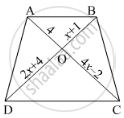Share

Books Shortlist
Your shortlist is empty

# Solution for In Below Figure, If Ab || Cd, Find the Value of X. - CBSE Class 10 - Mathematics

#### Question

In below figure, If AB || CD, find the value of x.#### Solution

Since diagonals of a trapezium divide each other proportionally.

therefore"AO"/"OC"="BO"/"OD"

rArr4/(4x-2)=(x+1)/(2x+4)

⇒ 4(2𝑥 + 4) = (𝑥 + 1)(4𝑥 − 2)

⇒ 8x + 16 = x(4x – 2) +1(4x – 2)

⇒ 8x + 16 = 4x2 + 2x – 2

⇒ 4x2 + 2𝑥 − 8𝑥 − 2 − 16 = 0

⇒ 4x2 − 6𝑥 − 18 = 0

⇒ 2[2𝑥2 − 3𝑥 − 9] = 0

⇒ 2𝑥2 − 3𝑥 − 9 = 0

⇒ 2𝑥(𝑥 − 3) + 3(𝑥 − 3) = 0

⇒ (𝑥 − 3)(2𝑥 + 3) = 0

⇒ 𝑥 − 3 = 0 or 2𝑥 + 3 = 0

⇒ 𝑥 = 3 or 𝑥 = -3/2

𝑥 = -3/2 is not possible, because

"OB"=x+1=-3/2+1=-1/2

Length cannot be negative

therefore"AO"/"OC"="BO"/"OD"

Is there an error in this question or solution?

#### Video TutorialsVIEW ALL 

Solution for question: In Below Figure, If Ab || Cd, Find the Value of X. concept: Triangles Examples and Solutions. For the course CBSE
S